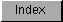```
After an application has successfully opened a library, it can start using
the library's functions.  To access a library function, an application
needs the library base address returned by OpenLibrary() and the
function's Library Vector Offset (LVO).  A function's LVO is the offset
from the library's base address to the function's vector in the vector
table, which means an LVO is a negative number (the vectors precedes the
library base in memory).  Application code enters a library function by
doing a jump to subroutine (the 680x0 instruction JSR) to the proper
negative offset (LVO) from the address of the library base.  The library
vector itself is a jump instruction (the 680x0 instruction JMP) to the
actual library function which is somewhere in memory.

Low Memory
/|\
|
________________|________________
|                                 |
|   JMP Function N (LVO=-(N*6))   |
|                ·                |
|                ·                |
|    JMP Function 6 (LVO=-36)     |
|    JMP Function 5 (LVO=-30)     |
| JMP Function Reserved (LVO=-24) |
| JMP Function Expunge (LVO=-18)  |
|  JMP Function Close (LVO=-12)   |
|   JMP Function Open (LVO=-6)    |
Library Base____|_________________________________|
|                                 |
|        Library Structure        |
|_________________________________|
|                                 |
|            Data Area            |
|_________________________________|
|
|
\|/
High Memory

Figure 17-1: An Exec Library Base in RAM

The only legal way for an application to access a library function is
through the vector table.  A function's LVO is always the same on every
system and is not subject to change.  A function's jump vector can, and
does, change.  Assuming that a function's jump vector is static is very
bad, so don't do it.

Each library has four vectors set aside for library housekeeping: OPEN,
CLOSE, EXPUNGE, and RESERVED.  The OPEN vector points to a function that
performs any custom initialization that this library needs, for example,
opening other libraries that this library uses.  The CLOSE function takes
care of any clean up necessary when an application closes a library.  The
EXPUNGE vector points to a function that prepares the library for removal
from the system.  Normally the OS will not remove a library from memory
until the system needs the memory the library occupies.  The other vector,
RESERVED, does not do anything at present and is reserved for future
system use.

```